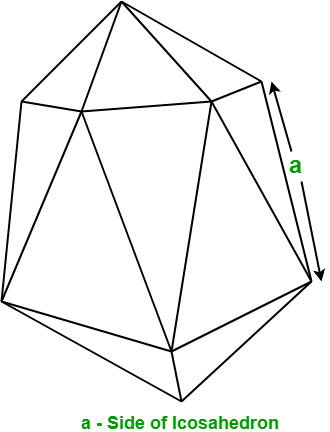# Program to find the Area and Volume of Icosahedron

Given the side of an Icosahedron. The task is to find the area and volume of the given Icosahedron.

Examples :

Input : a = 5
Output : Area: 216.506
Volume: 272.712

Input : a = 10
Output : Area: 866.0254
Volume: 2181.695


## Recommended: Please try your approach on {IDE} first, before moving on to the solution.

In geometry, an Icosahedron is a regular polyhedron which contains 20 identical equilateral triangular faces, 30 sides, and 12 vertices.Formula to find Area and Volume of Icosahedron: Let a be the side of Icosahedron, then

Surface area of Icosahedron =and, Volume of Icosahedron =## C++

 // C++ program to find the Area and  // volume of Icosahedron  #include  using namespace std;     // Function to find area of Icosahedron  float findArea(float a)  {      float area;         // Formula to calculating area      area = 5 * sqrt(3) * a * a;             return area;  }     // Function to find volume of Icosahedron  float findVolume(float a)  {      float volume;         // Formula to calculating volume      volume = ((float)5 / 12) * (3 + sqrt(5)) * a * a * a;             return volume;  }     // Driver Code  int main()  {      float a = 5;         // Function call to find area of Icosahedron.      cout << "Area: " << findArea(a) << endl;             // Function call to find volume of Icosahedron.      cout << "Volume: " << findVolume(a);         return 0;  }

## Java

 // Java program to find the Area and  // volume of Icosahedron  import java.io.*;     class GFG {             // Function to find area of Icosahedron      static float findArea(float a)      {          float area;                 // Formula to calculating area          area = (float)(5 * Math.sqrt(3) * a * a);                     return area;      }             // Function to find volume of Icosahedron      static float findVolume(float a)      {          float volume;                 // Formula to calculating volume          volume = (float)(((float)5 / 12) * (3 + Math.sqrt(5)) * a * a * a);                     return volume;      }             // Driver code      public static void main (String[] args)      {          float a = 5;             // Function call to find area of Icosahedron.          System.out.println("Area: " + findArea(a));                     // Function call to find volume of Icosahedron.          System.out.println("Volume: " + findVolume(a));      }  }

## Python3

 # Python3 program to   # find the Area and  # volume of Icosahedron     # import math module   # to use sqrt function  from math import sqrt     # Function to find   # area of Icosahedron  def findArea(a):         # Formula to calculate area      area = 5 * sqrt(3) * a * a      return area     # Function to find   # volume of Icosahedron  def findVolume(a):             # Formula to calculate volume      volume = ((5 / 12) *                (3 + sqrt(5)) *                 a * a * a)      return volume     # Driver Code  a = 5    # Function call to   # find area of Icosahedron.  print("Area: " , findArea(a))         # Function call to find  # volume of Icosahedron.  print("Volume: " , findVolume(a))     # This code is contributed  # by ihritik

## C#

 // C# program to find the Area and  // volume of Icosahedron  using System;     public class GFG {             // Function to find area of Icosahedron      static float findArea(float a)      {          float area;                 // Formula to calculating area          area = (float)(5 * Math.Sqrt(3) * a * a);                     return area;      }             // Function to find volume of Icosahedron      static float findVolume(float a)      {          float volume;                 // Formula to calculating volume          volume = (float)(((float)5 / 12) * (3 + Math.Sqrt(5)) * a * a * a);                     return volume;      }             // Driver code      static public void Main ()      {          float a = 5;             // Function call to find area of Icosahedron.          Console.WriteLine("Area: " + findArea(a));                     // Function call to find volume of Icosahedron.          Console.WriteLine("Volume: " + findVolume(a));          //Code      }  }

## PHP

 

Output:

Area: 216.506
Volume: 272.712


Don’t stop now and take your learning to the next level. Learn all the important concepts of Data Structures and Algorithms with the help of the most trusted course: DSA Self Paced. Become industry ready at a student-friendly price.

My Personal Notes arrow_drop_upCheck out this Author's contributed articles.

If you like GeeksforGeeks and would like to contribute, you can also write an article using contribute.geeksforgeeks.org or mail your article to contribute@geeksforgeeks.org. See your article appearing on the GeeksforGeeks main page and help other Geeks.

Please Improve this article if you find anything incorrect by clicking on the "Improve Article" button below.

Improved By : jit_t, ihritik

Article Tags :
Practice Tags :

Be the First to upvote.

Please write to us at contribute@geeksforgeeks.org to report any issue with the above content.